# Perfectly Contractile Graphs and the Decomposition Method

The following conjecture, due to Everett and Reed attempts to characterize perfectly contractile graphs.

Perfectly Contractile Graph Conjecture (PCGC) A graph is perfectly contractile if and only if it does not contain an odd hole, an antihole nor an odd prism.

We propose to investigate the PCGC and a possible construction of a polynomial-time recognition algorithm for perfectly contractile graphs, through the decomposition method.

The decomposition method is based on a decomposition theorem of the following form, for the class of graphs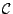we want to analyse.

Decomposition Theorem If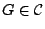, then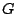is either basic or it contains certain types of cutsets.

Basic stands for a certain simple'' subclass of.

The idea of a decomposition based recognition algorithm for the classis as follows. In a connected graph, a node set (or an edge set or a combination of the two) is a cutset if its removal disconnectsinto two or more connected components. From these components blocks of decomposition are constructed by adding some more nodes and edges. A decomposition is-preserving if it satisfies the following:belongs toif and only if all the blocks of decomposition belong to. A decomposition based recognition algorithm takes an input graphand decomposes it using-preserving decompositions into a polynomial number of basic blocks, which are then checked, in polynomial time, whether they belong to.

Such a construction of blocks works nicely for clique cutsets. A node set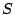is a star cutset of a graphif its removal disconnectsandcontains a node that is adjacent to all the other nodes of. With the usual construction of blocks for the node cutsets, the star cutset decomposition is not preserving for the class of perfectly contractile graphs.

A generalization of star cutsets is obtained as follows. 1-Amalgams are defined and used in for the construction of a recognition algorithm for Meyniel graphs. A graphhas a 1-amalgam if its vertex set can be partitioned into sets,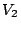and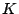(whereis possibly empty) in such a way that:

• for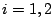,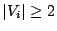and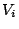contains a nonempty set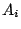;
• every node of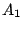is adjacent to every node of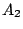and these are the only adjacencies between the nodes ofand the nodes of; and
• if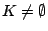, then it induces a clique, and every node ofis adjacent to every node of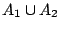.

A graphhas a 2-join if its node set can be partitioned into setsandso that for,contains disjoint nonempty setsand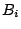, and the following properties hold:

• every node of(resp.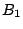) is adjacent to every node of(resp.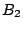), and these are the only adjacencies between the nodes ofand the nodes of;
• for, let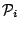be the set of all chordless paths in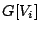with one endnode in, the other endnode in, and no intermediate node in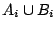. For,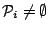andis not isomorphic to a path in.

Let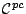denote the class of perfectly contractile graphs.

We have the following conjectures.

Conjecture 1-Amalgam decomposition is-preserving.

Conjecture 2-Join decomposition is-preserving.

Conjecture No minimal non perfectly contractile graph has a star cutset.

Note that the PCGC implies all three of these conjectures.

Contributed by Claudia Linhares Sales.

Back to the main index for Perfect Graphs.